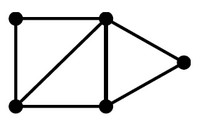# Graph - Data Structure (Physical Representation)

A graph is represented generally in a physical data structure

## Format

### Two set

The graph is composed of two set.

Node
a
b
c
a b
a c
b a
b c
c a
c b

The graph is composed of set.

 Node a b c
a b,c
b a,c
c a,b

Adjacency matrix is a matrix of adjacent vertices where the coordinates (x,y) may be stored in a set

a b c
a 0 1 1
b 1 0 1
c 1 1 1

## Documentation / Reference

Discover MoreGraph (Network - Nodes and edges)

A graph is a set of vertices connected by edges. See Data representation that naturally captures complex relationships is a graph (or network). Except of the special graph that a tree is, the data...Logical Data Modeling - Data Structure

A data structure is a single data instance that: cannot be compared/represented by a single value have operations that follows rules represents an entity, a relationship or both. has two representations:...